"Mean-Field Variational Bayes" (MFVB), is similar to expectation-maximization (EM) yet distinct in two key ways:

1. We do not minimize $$\text{KL}\big(q(\mathbf{Z})\Vert p(\mathbf{Z}\vert\mathbf{X}, \theta)\big)$$, i.e. perform the E-step, as [in the problems in which we employ mean-field] the posterior distribution $$p(\mathbf{Z}\vert\mathbf{X}, \theta)$$ "is too complex to work with,"™ i.e. it has no analytical form.
2. Our variational distribution $$q(\mathbf{Z})$$ is a factorized distribution, i.e.
$$q(\mathbf{Z}) = \prod\limits_i^{M} q_i(\mathbf{Z}_i)$$

for all latent variables $$\mathbf{Z}_i$$.

Briefly, factorized distributions are cheap to compute: if each $$q_i(\mathbf{Z}_i)$$ is Gaussian, $$q(\mathbf{Z})$$ requires optimization of $$2M$$ parameters (a mean and a variance for each factor); conversely, a non-factorized $$q(\mathbf{Z}) = \text{Normal}(\mu, \Sigma)$$ would require optimization of $$M$$ parameters for the mean and $$\frac{M^2 + M}{2}$$ parameters for the covariance. Following intuition, this gain in computational efficiency comes at the cost of decreased accuracy in approximating the true posterior over latent variables.

## So, what is it?

Mean-field Variational Bayes is an iterative maximization of the ELBO. More precisely, it is an iterative M-step with respect to the variational factors $$q_i(\mathbf{Z}_i)$$.

In the simplest case, we posit a variational factor over every latent variable, as well as every parameter. In other words, as compared to the log-marginal decomposition in EM, $$\theta$$ is absorbed into $$\mathbf{Z}$$.

$$\log{p(\mathbf{X}\vert\theta)} = \mathop{\mathbb{E}}_{q(\mathbf{Z})}\bigg[\log{\frac{p(\mathbf{X, Z}\vert\theta)}{q(\mathbf{Z})}}\bigg] + \text{KL}\big(q(\mathbf{Z})\Vert p(\mathbf{Z}\vert\mathbf{X}, \theta)\big)\quad \text{(EM)}$$

becomes

$$\log{p(\mathbf{X})} = \mathop{\mathbb{E}}_{q(\mathbf{Z})}\bigg[\log{\frac{p(\mathbf{X, Z})}{q(\mathbf{Z})}}\bigg] + \text{KL}\big(q(\mathbf{Z})\Vert p(\mathbf{Z}\vert\mathbf{X})\big)\quad \text{(MFVB)}$$

From there, we simply maximize the ELBO, i.e. $$\mathop{\mathbb{E}}_{q(\mathbf{Z})}\bigg[\log{\frac{p(\mathbf{X, Z})}{q(\mathbf{Z})}}\bigg]$$, by iteratively maximizing with respect to each variational factor $$q_i(\mathbf{Z}_i)$$ in turn.

## What's this do?

Curiously, we note that $$\log{p(\mathbf{X})}$$ is a fixed quantity with respect to $$q(\mathbf{Z})$$: updating our variational factors will not change the marginal log-likelihood of our data.

This said, we note that the ELBO and $$\text{KL}\big(q(\mathbf{Z})\Vert p(\mathbf{Z}\vert\mathbf{X})\big)$$ trade off linearly: when one goes up by $$\Delta$$, the other goes down by $$\Delta$$.

As such, (iteratively) maximizing the ELBO in MFVB is akin to minimizing the divergence between the true posterior over the latent variables given data and our factorized variational approximation thereof.

## Derivation

So, what do these updates look like?

First, let's break the ELBO into its two main components:

\begin{align*} \mathop{\mathbb{E}}_{q(\mathbf{Z})}\bigg[\log{\frac{p(\mathbf{X, Z})}{q(\mathbf{Z})}}\bigg] &= \int{q(\mathbf{Z})\log{\frac{p(\mathbf{X, Z})}{q(\mathbf{Z})}}}d\mathbf{Z}\\ &= \int{q(\mathbf{Z})\log{p(\mathbf{X, Z})}}d\mathbf{Z} - \int{q(\mathbf{Z})\log{q(\mathbf{Z})}}d\mathbf{Z}\\ &= A + B \end{align*}

Next, rewrite this expression in a way that isolates a single variational factor $$q_j(\mathbf{Z}_j)$$, i.e. the factor with respect to which we'd like to maximize the ELBO in a given iteration.

## Expanding the first term

\begin{align*} A &= \int{q(\mathbf{Z})\log{p(\mathbf{X, Z})}d\mathbf{Z}}\\ &= \int{\prod\limits_{i}q_i(\mathbf{Z}_i)\log{p(\mathbf{X, Z})}d\mathbf{Z}_i}\\ &= \int{q_j(\mathbf{Z}_j)\bigg[\int{\prod\limits_{i \neq j}q_i(\mathbf{Z}_{i})\log{p(\mathbf{X, Z})}}d\mathbf{Z}_i\bigg]}d\mathbf{Z}_j\\ &= \int{q_j(\mathbf{Z}_j){ \mathop{\mathbb{E}}_{i \neq j}[\log{p(\mathbf{X, Z})}] }d\mathbf{Z}_j}\\ \end{align*}

Following Bishop1's derivation, we've introduced the notation:

$$\int{\prod\limits_{i \neq j}q_i(\mathbf{Z}_{i})\log{p(\mathbf{X, Z})}}d\mathbf{Z}_i = \mathop{\mathbb{E}}_{i \neq j}[\log{p(\mathbf{X, Z})}]$$

A few things to note, and in case this looks strange:

• Were the left-hand side to read $$\int{q(\mathbf{Z})\log{p(\mathbf{X, Z})}}d\mathbf{Z}$$, this would look like the perfectly vanilla expectation $$\mathop{\mathbb{E}}_{q(\mathbf{Z})}[\log{p(\mathbf{X, Z})}]$$.
• An expectation maps a function $$f$$, e.g. $$\log{p(\mathbf{X, Z})}$$, to a single real number. As our expression reads $$\mathop{\mathbb{E}}_{i \neq j}[\log{p(\mathbf{X, Z})}]$$ as opposed to $$\mathop{\mathbb{E}}_{q(\mathbf{Z})}[\log{p(\mathbf{X, Z})}]$$, we're conspicuously unable to integrate over the remaining factor $$q_j(\mathbf{Z}_j)$$
• As such, $$\mathop{\mathbb{E}}_{i \neq j}[\log{p(\mathbf{X, Z})}]$$ gives a function of the value of $$\mathbf{Z}_j$$ which itself maps to the aforementioned real number.

To further illustrate, let's employ some toy Python code:

# Suppose Z = [Z_0, Z_1, Z_2], with corresponding (discrete) variational distributions q_0, q_1, q_2

q_0 = [
(1, .2),  # q_0(1) = .2
(2, .3),
(3, .5)
]

q_1 = [
(4, .3),
(5, .3),
(6, .4)
]

q_2 = [
(7, .7),
(8, .2),
(9, .1)
]

dists = (q_0, q_1, q_2)

# Next, suppose we'd like to isolate Z_2
j = 2


$$\mathop{\mathbb{E}}_{i \neq j}[\log{p(\mathbf{X, Z})}]$$, written E_i_neq_j_log_p_X_Z below, can be computed as:

def E_i_neq_j_log_p_X_Z(Z_j):

E = 0
Z_i_neq_j_dists = [dist for i, dist in enumerate(dists) if i != j]

for comb in product(*Z_i_neq_j_dists):
Z = []
prob = 0
comb = list(comb)
for i in range(len(dists)):
if i == j:
Z.append(Z_j)
else:
Z_i, p = comb.pop(0)
Z.append(Z_i)
if prob == 0:
prob = p
else:
prob *= p
E += prob * ln_p_X_Z(X, Z)

return E

• Continuing with our notes, it was not immediately obvious to me how and why we're able to introduce a second integral sign on line 3 of the derivation above. Notwithstanding, the reason is quite simple; a simple exercise of nested for-loops is illustrative.

Before beginning, we remind the definition of an integral in code. In its simplest example, $$\int{ydx}$$ can be written as:

x = np.linspace(lower_lim, upper_lim, n_ticks)

integral = sum([y * dx for dx in x])

# ...where n_ticks approaches infinity.


With this in mind, the following confirms the self-evidence of the second integral sign:

X = np.array([10, 20, 30])

def ln_p_X_Z(X, Z):
return (X + Z).sum()  # some dummy expression

# Line 2 of Expanding the first term
total = 0
for Z_0 in q_0:
for Z_1 in q_1:
for Z_2 in q_2:
val_z_0, prob_z_0 = Z_0
val_z_1, prob_z_1 = Z_1
val_z_2, prob_z_2 = Z_2
Z = np.array([val_z_0, val_z_1, val_z_2])
total += prob_z_0 * prob_z_1 * prob_z_2 * ln_p_X_Z(X, Z)

TOTAL = total

# Line 3 of Expanding the first term
total = 0
for Z_0 in q_0:
_total = 0
val_z_0, prob_z_0 = Z_0
for Z_1 in q_1:
for Z_2 in q_2:
val_z_1, prob_z_1 = Z_1
val_z_2, prob_z_2 = Z_2
Z = np.array([val_z_0, val_z_1, val_z_2])
_total += prob_z_1 * prob_z_2 * ln_p_X_Z(X, Z)
total += prob_z_0 * _total

assert total == TOTAL


In effect, isolating $$q_j(\mathbf{Z}_j)$$ is akin to the penultimate line total += prob_z_0 * _total, i.e. multiplying $$q_j(\mathbf{Z}_j)$$ by an intermediate summation _total. Therefore, the second integral sign is akin to _total += prob_z_1 * prob_z_2 * ln_p_X_Z(X, Z), i.e. the computation of this intermediate summation itself.

More succinctly, a multi-dimensional integral can be thought of as a nested-for-loop which commutes a global sum. Herein, we are free to compute intermediate sums at will.

## Expanding the second term

Next, let's expand $$B$$. We note that this is the entropy of the full variational distribution $$q(\mathbf{Z})$$.

\begin{align*} B &= - \int{q(\mathbf{Z})\log{q(\mathbf{Z})}}d\mathbf{Z}\\ &= - \mathop{\mathbb{E}}_{q(\mathbf{Z})}\bigg[\log{q(\mathbf{Z})}\bigg]\\ &= - \mathop{\mathbb{E}}_{q(\mathbf{Z})}\bigg[\log{\prod\limits_{i}q_i(\mathbf{Z}_i)}\bigg]\\ &= - \mathop{\mathbb{E}}_{q(\mathbf{Z})}\bigg[\sum\limits_{i}\log{q_i(\mathbf{Z}_i)}\bigg]\\ &= - \mathop{\mathbb{E}}_{q(\mathbf{Z})}\bigg[\log{q_j(\mathbf{Z}_j)} + \sum\limits_{i \neq j}\log{q_i(\mathbf{Z}_i)}\bigg]\\ &= - \mathop{\mathbb{E}}_{q(\mathbf{Z})}\bigg[\log{q_j(\mathbf{Z}_j)}\bigg] - \mathop{\mathbb{E}}_{q(\mathbf{Z})}\bigg[\sum\limits_{i \neq j}\log{q_i(\mathbf{Z}_i)}\bigg]\\ &= - \mathop{\mathbb{E}}_{q_j(\mathbf{Z}_j)}\bigg[\log{q_j(\mathbf{Z}_j)}\bigg] - \mathop{\mathbb{E}}_{q_{i \neq j}(\mathbf{Z}_i)}\bigg[\sum\limits_{i \neq j}\log{q_i(\mathbf{Z}_i)}\bigg]\\ &= - \mathop{\mathbb{E}}_{q_j(\mathbf{Z}_j)}\bigg[\log{q_j(\mathbf{Z}_j)}\bigg] + \text{const}\\ &= - \int{q_j(\mathbf{Z}_j)\log{q_j(\mathbf{Z}_j)}}d\mathbf{Z}_j + \text{const}\\ \end{align*}

As we'll be maximizing w.r.t. just $$q_j(\mathbf{Z}_j)$$, we can set all terms that don't include this factor to constants.

## Putting it back together

\begin{align*} \mathop{\mathbb{E}}_{q(\mathbf{Z})}\bigg[\log{\frac{p(\mathbf{X, Z})}{q(\mathbf{Z})}}\bigg] &= A + B\\ &= \int{q_j(\mathbf{Z}_j){ \mathop{\mathbb{E}}_{i \neq j}[\log{p(\mathbf{X, Z})}] }d\mathbf{Z}_j} - \int{q_j(\mathbf{Z}_j)\log{q_j(\mathbf{Z}_j)}}d\mathbf{Z}_j + \text{const}\\ \end{align*}

## One final pseudonym

Were we able to replace the expectation in $$A$$ with the $$\log$$ of some density $$D$$, i.e.

$$= \int{q_j(\mathbf{Z}_j){ \log{D} }\ d\mathbf{Z}_j} - \int{q_j(\mathbf{Z}_j)\log{q_j(\mathbf{Z}_j)}}d\mathbf{Z}_j + \text{const}$$

$$A + B$$ could be rewritten as $$-\text{KL}(q_j(\mathbf{Z}_j)\Vert D)$$.

Acknowledging that $$\mathop{\mathbb{E}}_{i \neq j}[\log{p(\mathbf{X, Z})}]$$ is an unnormalized log-likelihood written as a function of $$\mathbf{Z}_j$$, we temporarily rewrite it as:

$$\mathop{\mathbb{E}}_{i \neq j}[\log{p(\mathbf{X, Z})}] = \log{\tilde{p}(\mathbf{X}, \mathbf{Z}_j})$$

As such:

\begin{align*} \mathop{\mathbb{E}}_{q(\mathbf{Z})}\bigg[\log{\frac{p(\mathbf{X, Z})}{q(\mathbf{Z})}}\bigg] &= \int{q_j(\mathbf{Z}_j){ \log{\tilde{p}(\mathbf{X}, \mathbf{Z}_j}) }d\mathbf{Z}_j} - \int{q_j(\mathbf{Z}_j)\log{q_j(\mathbf{Z}_j)}}d\mathbf{Z}_j + \text{const}\\ &= \int{q_j(\mathbf{Z}_j){ \log{\frac{\tilde{p}(\mathbf{X}, \mathbf{Z}_j)}{q_j(\mathbf{Z}_j)}} }d\mathbf{Z}_j} + \text{const}\\ &= - \text{KL}\big(q_j(\mathbf{Z}_j)\Vert \tilde{p}(\mathbf{X}, \mathbf{Z}_j)\big) + \text{const}\\ \end{align*}

Finally, per this expression, the ELBO reaches its minimum when:

\begin{align*} q_j(\mathbf{Z}_j) &= \tilde{p}(\mathbf{X}, \mathbf{Z}_j)\\ &= \exp{\bigg(\mathop{\mathbb{E}}_{i \neq j}[\log{p(\mathbf{X, Z})}]\bigg)} \end{align*}

Or equivalently:

$$\log{q_j(\mathbf{Z}_j)} = \mathop{\mathbb{E}}_{i \neq j}[\log{p(\mathbf{X, Z})}]$$

Summing up:

• Iteratively minimizing the divergence between $$q_j(\mathbf{Z}_j)$$ and $$\tilde{p}(\mathbf{X}, \mathbf{Z}_j)$$ for all factors $$j$$ is our mechanism for maximizing the ELBO
• In turn, maximizing the ELBO is our mechanism for minimizing the KL divergence between the full factorized posterior $$q(\mathbf{Z})$$ and the true posterior $$p(\mathbf{Z}\vert\mathbf{X})$$.

Finally, as the optimal density $$q_j(\mathbf{Z}_j)$$ relies on those of $$q_{i \neq j}(\mathbf{Z}_{i})$$, this optimization algorithm is necessarily iterative.

## Normalization constant

Nearing the end, we note that $$q_j(\mathbf{Z}_j) = \exp{\bigg(\mathop{\mathbb{E}}_{i \neq j}[\log{p(\mathbf{X, Z})}]\bigg)}$$ is not necessarily a normalized density (over $$\mathbf{Z}_j$$). "By inspection," we compute:

\begin{align*} q_j(\mathbf{Z}_j) &= \frac{\exp{\bigg(\mathop{\mathbb{E}}_{i \neq j}[\log{p(\mathbf{X, Z})}]\bigg)}}{\int{\exp{\bigg(\mathop{\mathbb{E}}_{i \neq j}[\log{p(\mathbf{X, Z})}]\bigg)}d\mathbf{Z}_j}}\\ &= \exp{\bigg(\mathop{\mathbb{E}}_{i \neq j}[\log{p(\mathbf{X, Z})}]\bigg)} + \text{const}\\ \end{align*}

## How to actually employ this thing

First, plug in values for the right-hand side of:

$$\log{q_j(\mathbf{Z}_j)} = \mathop{\mathbb{E}}_{i \neq j}[\log{p(\mathbf{X, Z})}]$$

Then, attempt to rearrange this expression such that:

Once exponentiated, giving $$\exp{\big(\log{q_j(\mathbf{Z}_j)}\big)} = q_j(\mathbf{Z}_j)$$, we are left with something that, once normalized (by inspection), resembles a known density function (e.g. a Gaussian, a Gamma, etc.).

NB: This may require significant computation.

# Approximating a Gaussian

Here, we'll approximate a 2D multivariate Gaussian with a factorized mean-field approximation.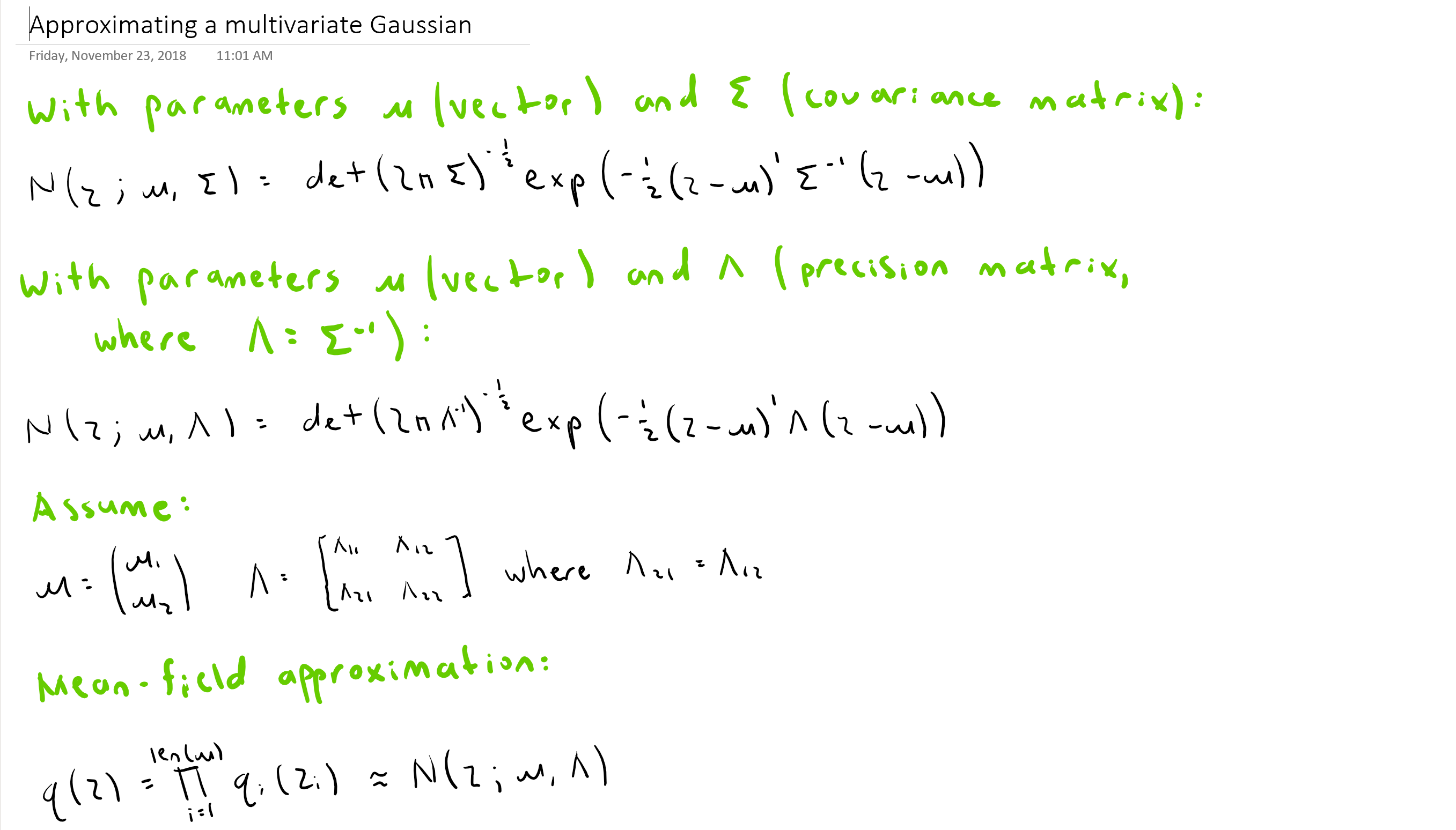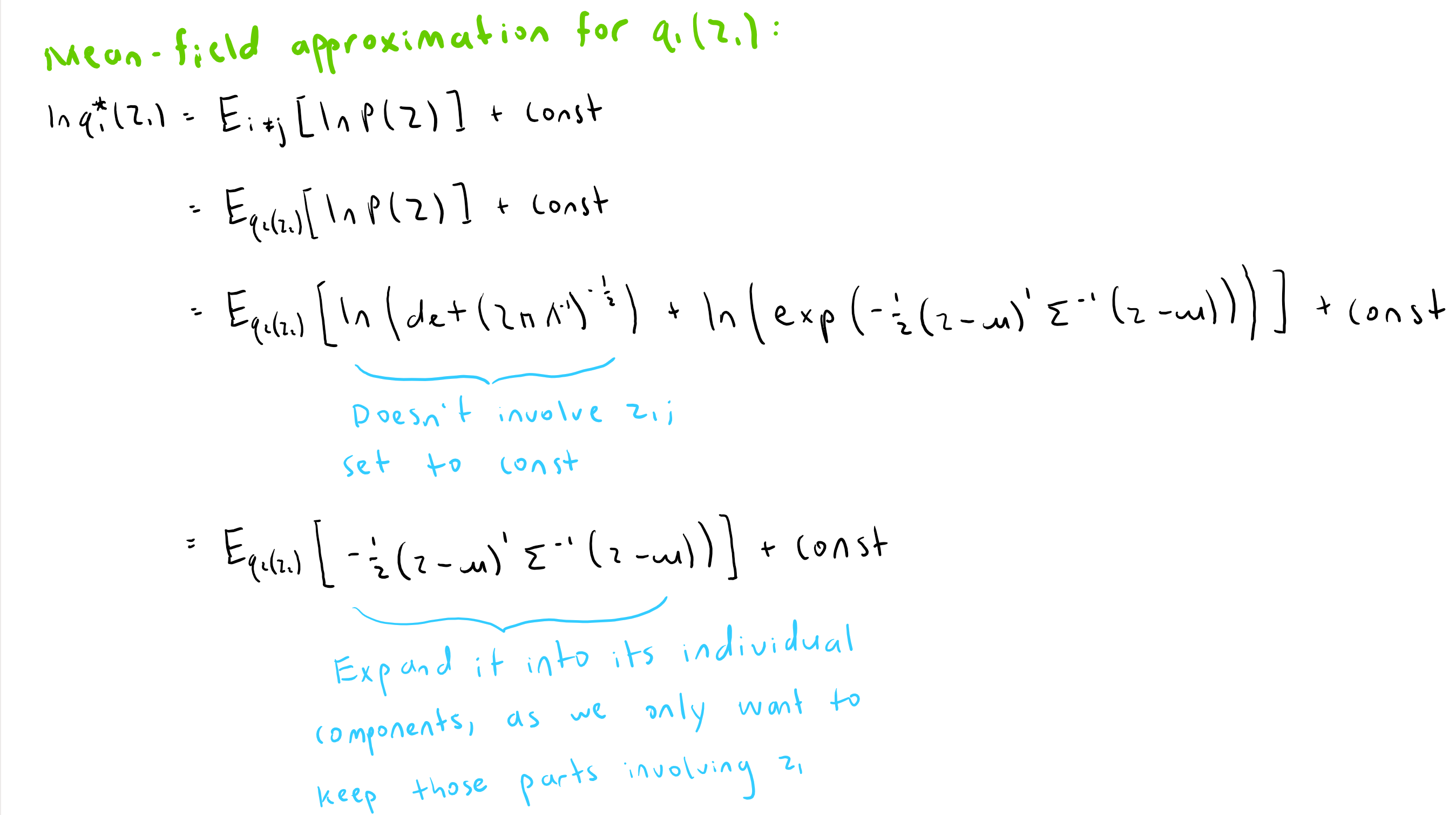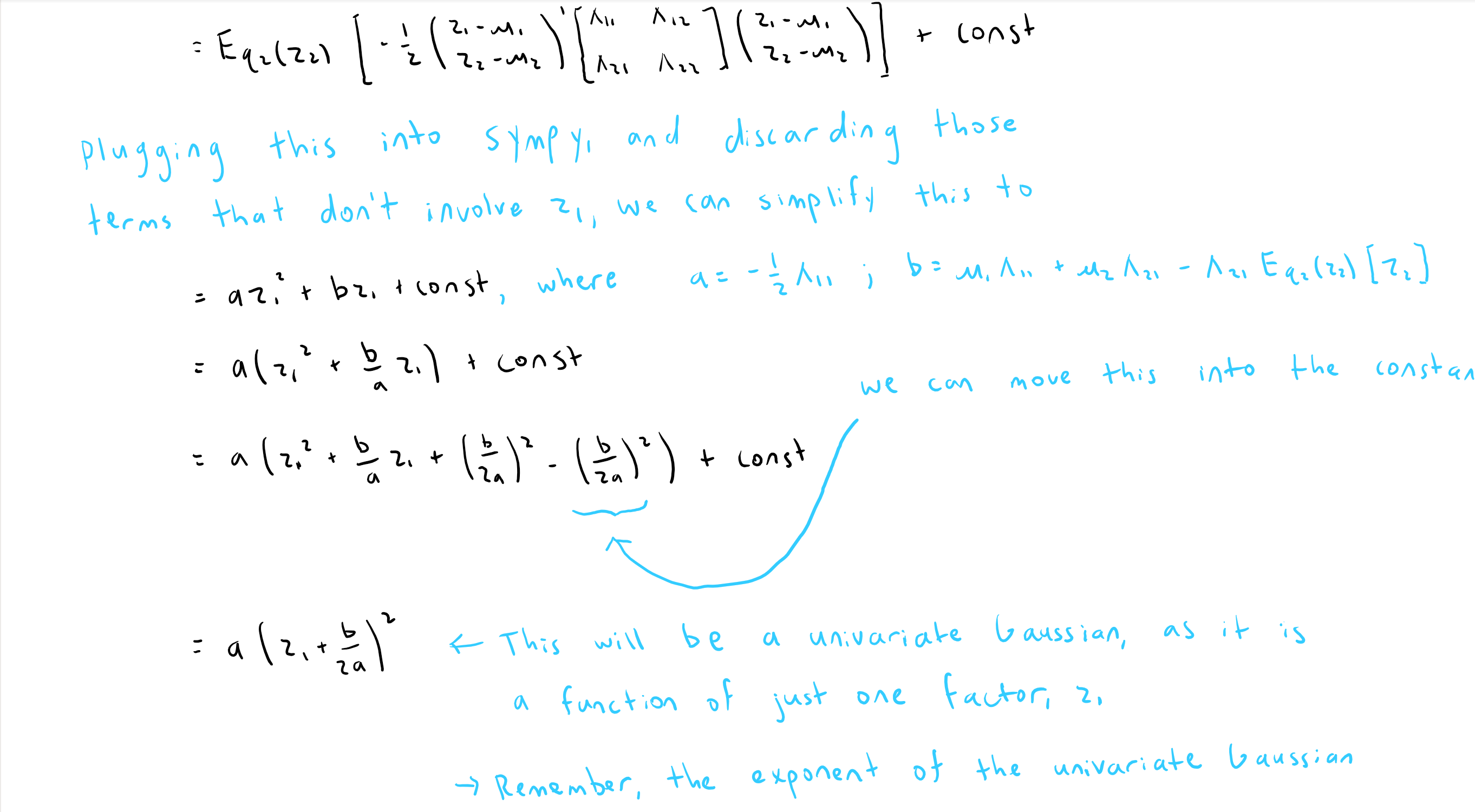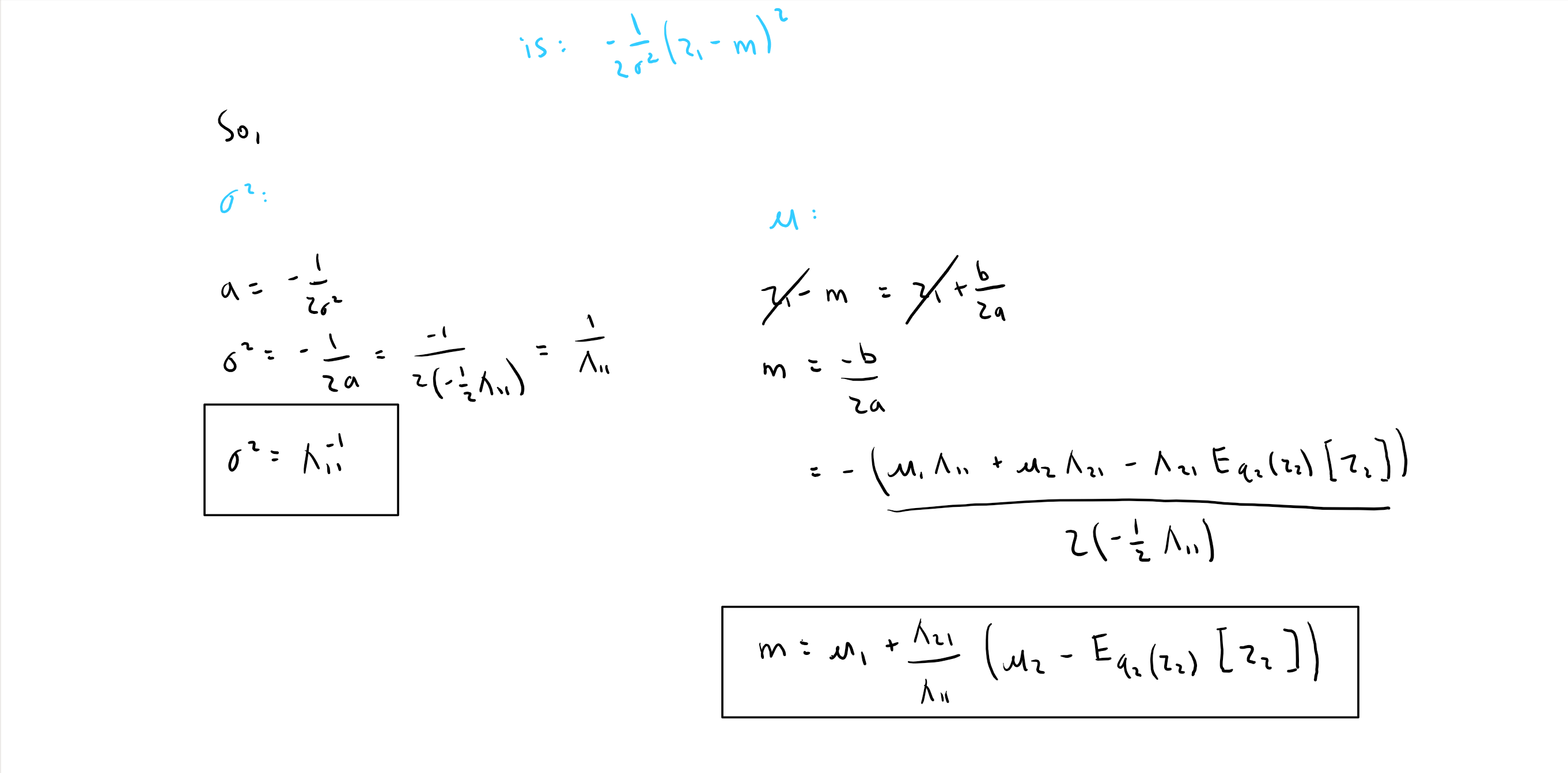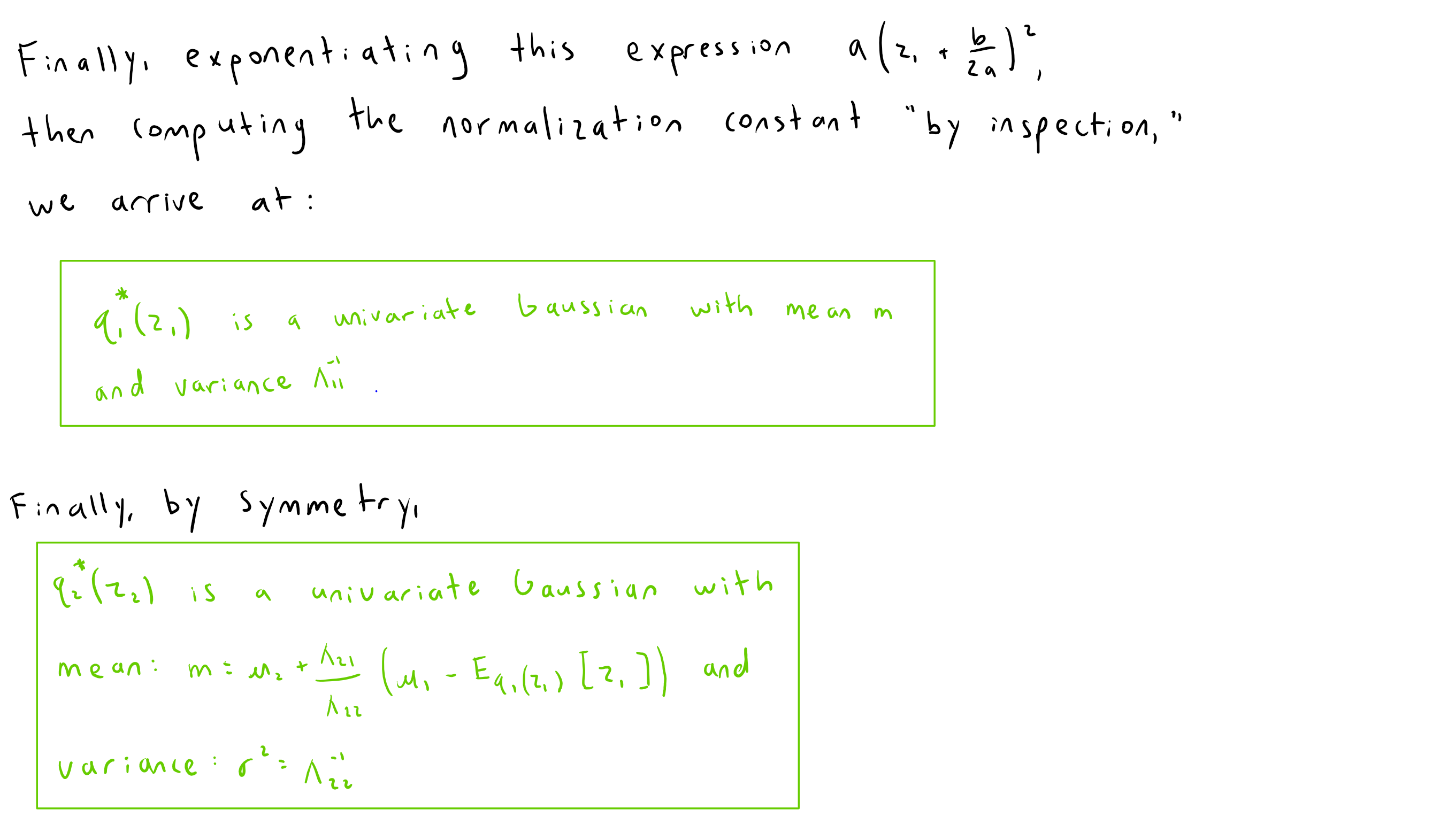# Summing up

Mean-Field Variational Bayes is an iterative optimization algorithm for maximizing a lower-bound of the marginal likelihood of some data $$\mathbf{X}$$ under a given model with latent variables $$\mathbf{Z}$$. It accomplishes this task by positing a factorized variational distribution over all latent variables $$\mathbf{Z}$$ and parameters $$\theta$$, then computes, analytically, the algebraic forms and parameters of each factor which maximize this bound.

In practice, this process can be cumbersome and labor-intensive. As such, in recent years, "black-box variational inference" techniques were born, which fix the forms of each factor $$q_j(\mathbf{Z}_j)$$, then optimize its parameters via gradient descent.

## References

1. C. M. Bishop. Pattern recognition and machine learning, page 229. Springer-Verlag New York, 2006.